# The UCM Procedure

### SEASON Statement

• SEASON LENGTH = integer <options>;

The SEASON or SEASONAL statement is used to specify a seasonal component,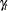, in the model. A seasonal component can be one of the two types, DUMMY or TRIG. A DUMMY seasonal with season length s satisfies the following stochastic equation: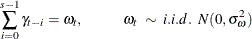The equations for a TRIG (short for trigonometric) seasonal component are as follows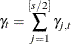where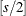equals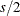if s is even and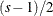if it is odd. The sinusoids, also called harmonics,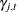have frequencies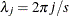and are specified by the matrix equation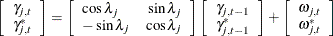where the disturbances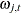and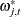are assumed to be independent and, for fixed j,and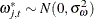. If s is even, then the equation for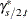is not needed and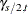is given by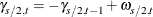In the TRIG seasonal case, the option KEEPH= or DROPH= can be used to obtain subset trigonometric seasonals that contain only a subset of the full set of harmonics,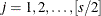. This is particularly useful when the season length s is large and the seasonal pattern is relatively smooth.

Note that whether the seasonal type is DUMMY or TRIG, there is only one parameter, the disturbance variance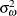, in the seasonal model.

There can be more than one seasonal component in the model, necessarily with different season lengths if the seasons are full. You can have multiple subset season components with the same season length, if you need to use separate disturbance variances for different sets of harmonics. Each seasonal component is specified using a separate SEASON statement. A model with multiple seasonal components can easily become quite complex and might need a large amount of data and computing resources for its estimation and forecasting. The examples that follow illustrate the use of SEASON statement.

The following statement specifies a DUMMY type (default) seasonal component with a season length of four, corresponding to the quarterly seasonality. The disturbance varianceis estimated from the data.

      season length=4;


The following statement specifies a trigonometric seasonal with monthly seasonality. It also provides a starting value for.

      season length=12 type=trig variance=4;

DROPHARMONICS|DROPH=number-list |  n TO m BY p

enables you to drop some harmonicsfrom the full set of harmonics used to obtain a trigonometric seasonal. The drop list can include any integer between 1 and, s being the season length. For example, the following specification results in a specification of a trigonometric seasonal with a season length 12 that consists of only the first four harmonics,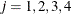:

      season length=12 type=trig DROPH=5 6;


The last two high frequency harmonics are dropped. The DROPH= option cannot be used with the KEEPH= option.

KEEPHARMONICS|KEEPH=number-list |  n TO m BY p

enables you to keep only the harmonicslisted in the option to obtain a trigonometric seasonal. The keep list can include any integer between 1 and, s being the season length. For example, the following specification results in a specification of a trigonometric seasonal with a season length of 12 that consists of all the six harmonics,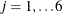:

      season length=12 type=trig KEEPH=1 to 3;
season length=12 type=trig KEEPH=4 to 6;


However, these six harmonics are grouped into two groups, each having its own disturbance variance parameter. The DROPH= option cannot be used with the KEEPH= option.

LENGTH=integer

specifies the season length, s. This is a required option in this statement. The season length can be any integer greater than or equal to 2. Typical examples of season lengths are 12, corresponding to the monthly seasonality, or 4, corresponding to the quarterly seasonality.

NOEST

fixes the value of the disturbance variance parameter to the value specified in the VARIANCE= option.

PLOT=FILTER
PLOT=SMOOTH
PLOT=F_ANNUAL
PLOT=S_ANNUAL
PLOT=( <plot request> …<plot request> )

requests plots of the season component. When you specify only one plot request, you can omit the parentheses around the plot request. You can use the FILTER and SMOOTH options to plot the filtered and smoothed estimates of the season component. You can use the F_ANNUAL and S_ANNUAL options to get the plots of "annual" variation in the filtered and smoothed estimates of. The annual plots are useful to see the change in the contribution of a particular month over the span of years. Here "month" and "year" are generic terms that change appropriately with the interval type being used to label the observations and the season length. For example, for monthly data with a season length of 12, the usual meaning applies, while for daily data with a season length of 7, the days of the week serve as months and the weeks serve as years.

PRINT=HARMONICS

requests printing of the summary of harmonics present in the seasonal component. This option is valid only for the trigonometric seasonal component.

PRINT=FILTER
PRINT=SMOOTH
PRINT=( <print request> …<print request> )

requests printing of the filtered or smoothed estimate of the seasonal component.

TYPE=DUMMY | TRIG

specifies the type of the seasonal component. The default type is DUMMY.

VARIANCE=value

specifies an initial value for the disturbance variance,, in theequation at the start of the parameter estimation process. Any nonnegative value, including zero, is an acceptable starting value.